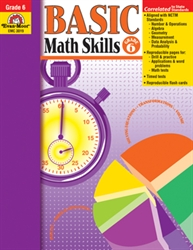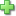# Basic Math Skills 6

Current Retail Price: \$29.99
Not in stock

See series description for full review.

In Basic Math Skills, Grade 6+, you’ll find just what you need to provide extra skill practice for individual students, small groups, or the whole class. These reproducible pages are perfect for homework too.

Skills practiced include:

Number and Operations

• Computation with whole numbers
• Computation with fractions
• Computation with decimals
• Percents and their equivalencies
• Number theory

Algebra

• Function tables
• Number lines and the coordinate system
• Order of operations
• Solving equations

Geometry

• Identify congruent shapes using transformational geometry
• Identify lines of symmetry in two-dimensional shapes
• Classify angles as acute, right, or obtuse
• Identify characteristics of three-dimensional solids

Measurement

• Customary and metric measurement
• Calculate elapsed time
• Measure angles using a protractor
• Perimeter, area, and volume

Data Analysis and Probability

• Construct and interpret graphs
• Analyze data using range, mean, median, and mode
• Compute theoretical probabilities for simple chance events
• Utilize counting techniques, tree charts, and organized lists to determine all possible combinations
Did you find this review helpful?
Series Description
Related Categories
Recommended for...
Customer ReviewsClick here to write a review